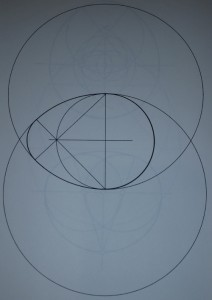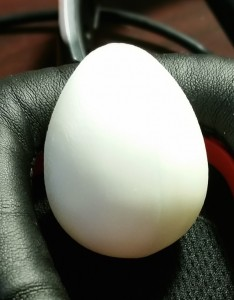# teaching machines

## Egg

In Mathographics, Robert Dixon tells me that any curve can be decomposed into a sequence of arcs. One can also go in reverse: lay out a bunch of intersecting circles, trace an arc on one circle, but then jump ship to another arc when you reach an intersection. If the circles intersect such that they also pass through each other’s origins, one traces curves that are egg-shaped. Here’s Dixon’s example of four circles (two big ones laid out vertically, a medium one in the center, and a quadrant of a small one on the left) and the egg that they generate:I implemented Dixon’s algorithm in Madeup and revolved the curve to lay a solid egg:My 3D printer only lays half-eggs at a time, but a little glue can put them back together:The Madeup source that produced this egg took a long time to get right. I didn’t have any arrays or vector functions builtin when I started.

to circsect a b
-- Finds the angle of the vector connecting
-- two circles' centers.
-- c1 and c2 are {center x, center y, radius}

-- What's the vector between the centers?
diff = b[0..1] - a[0..1]
a2b = normalize diff

-- How much is that vector rotated?
atan2 a2b, a2b
end

to arc c start stop
-- Draws an arc along circle c, starting at angle
-- start and ending at angle stop.
-- c is {center x, center y, radius}.
scale c, c, 1
translate c, c, 0

delta = (stop - start) // 20

current = start
while current <= stop
x = cos current
y = sin current
moveto x, y, 0
current = current + delta
end

identity
end

r1 = 2
r2 = 2 * r1
r3 = r2 - r1 * 2 ^ 0.5

-- Circle structs
c1 = {0, 0, r1} -- medium
c2 = {0, c1, r2} -- big top
c3 = {0, -c1, r2} -- big bottom
c4 = {-c1, 0, r3} -- small left

-- Find the angles for the circle pairs
angle12 = circsect c1, c2
angle31 = circsect c3, c1
angle34 = circsect c3, c4

-- Draw the piecewise curve
arc c1, 0, angle12
arc c3, angle31, angle34
arc c4, angle34, 180

-- Eliminate closies
coalesce 0.001

-- Spin the curve around to make an egg
nsides = 100
revolve 1, 0, 0, 180